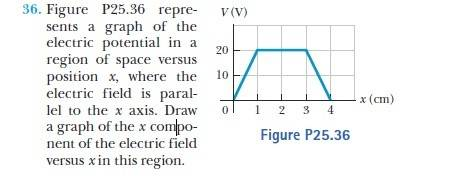# Electric potential graph analysis

freshcoast

## Homework StatementΔU = -q∫E * ds

## The Attempt at a Solution

Just by looking at the graph I am thinking, if I solve for E using the equation above, the number would be always negative therefore I can conclude that the electric field at any x position would be negative and the graph will look the same it will be just inverted. (-y values)

I don't know if this is the correct way to look at the problem or if I have to use energy conservation but any help would be much appreciated.

Thanks!

Last edited:

## Answers and Replies

Tanya Sharma
Ex = -dV/dx =negative of the slope of V-x graph.

What is the slope of graph between 0 and 1,1 and 3,3 and 4 ?

freshcoast
between 0 and 1, the slope is positive, 1 and 3 the slope is zero, 3 and 4 the slope is negative.

So if I use that equation you posted, between 0 and 1, the electric field will be negative, between 1 and 3 the electric field remains negative and then when x is between 3 and 4 the slope will be positive?

Tanya Sharma
between 0 and 1, the slope is positive, 1 and 3 the slope is zero, 3 and 4 the slope is negative.

So if I use that equation you posted, between 0 and 1, the electric field will be negative, between 1 and 3 the electric field remains negative and then when x is between 3 and 4 the slope will be positive?